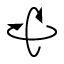# Parallel and skew lines

In the first activity, construct a plane that passes through points A, B, and D. definitions/distinctions: Parallel and skew lines share the characteristic that they both are pairs of lines that do not intersect. The difference is that parallel lines do not intersect and are contained on the same plane. Skew lines do not intersect but cannot be contained on the same plane. Now construct plane ABD by using thetool. Change the view by using thetool.

Does the plane you created contain points A, B, D, and E?

What can we conclude about lines AB and DE since they are contained on this new plane?

Select all that apply
• A
• B
• C
In the second activity, construct a plane that passes through points A, B, and E.

Does the plane you created contain all the points A, B, E, and F?

Construct a line parallel to line AB through point F. Drag the diagram around to see all three lines parallel to each other.

What can we conclude about lines AB and FE since they are not contained on this new plane?

Select all that apply
• A
• B
• C